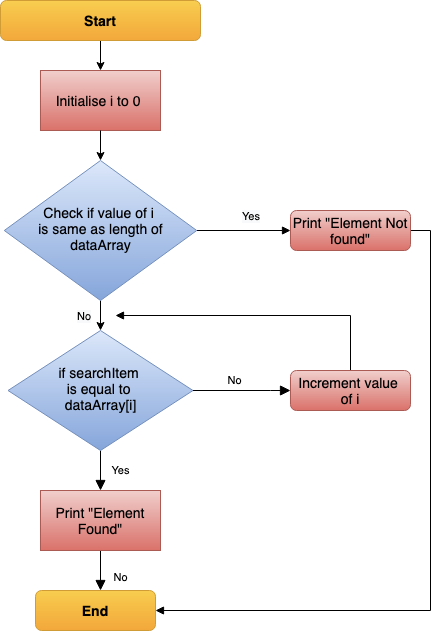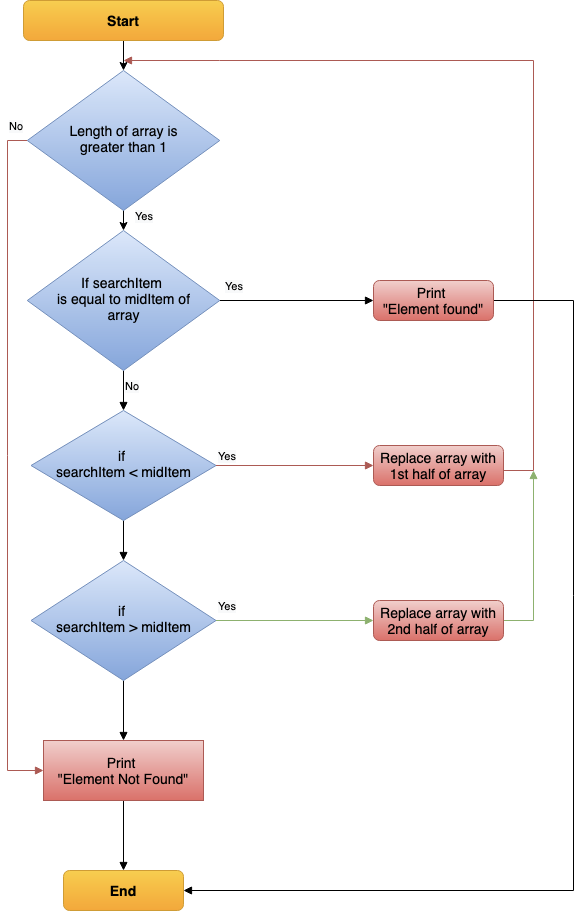1599645721

# Bubble Sort Algorithm Demystified

As Bubble Sort represents the basic foundations of sorting, we’ve tried to demystify this sorting algorithm.

#bubble-sort #sorting #sorting-algorithm #array #practice

## Buddha Community1599645721

## Bubble Sort Algorithm Demystified

As Bubble Sort represents the basic foundations of sorting, we’ve tried to demystify this sorting algorithm.

#bubble-sort #sorting #sorting-algorithm #array #practice1626753219

## Bubble Sort Algorithm | What Is Bubble Sort & How Bubble Sort Works? | Data Structures

This video is based on Bubble Sort Algorithm. In this Data structures tutorial, you will understand the fundamentals of sorting. This video is dedicated to helping beginners understand what is bubble sort algorithm & how bubble sort works in real-time. The video also includes a practical example for a better learning experience.

The video covers the following topics.

• 00:00:00 Introduction
• 00:01:04 What is a bubble sort algorithm?
• 00:02:03 How Does the Bubble Sort Algorithm Work?
• 00:06:09 Pseudocode of Bubble Sort Algorithm
• 00:06:44 Optimized Bubble Sort Algorithm
• 00:07:31 Variation oF Bubble Sort Algorithm
• 00:07:59 The complexity of the Bubble Sort Algorithm
• 00:09:28 Applications of Bubble Sort Algorithm
• 00:10:08 Demo

What Is a Data Structure?
The short answer is: a data structure is a specific means of organizing data in a system to access and use. The long answer is a data structure is a blend of data organization, management, retrieval, and storage, brought together into one format that allows efficient access and modification. It’s collecting data values, the relationships they share, and the applicable functions or operations.

Why Is Data Structure Important?
The digital world processes an increasing amount of data every year. According to Forbes, there are 2.5 quintillion bytes of data generated daily. The world created over 90 percent of the existing data in 2018 in the previous two years! The Internet of Things (IoT) is responsible for a significant part of this data explosion. Data structures are necessary to manage the massive amounts of generated data and a critical factor in boosting algorithm efficiency. Finally, since nearly all software applications use data structures and algorithms, your education path needs to include learning data structure and algorithms if you want a career as a data scientist or programmer. Interviewers want qualified candidates who understand how to use data structures and algorithms, so the more you know about the concepts, the more comfortably and confidently you will answer data structure interview questions.

#algorithm #data-structure #bubble1620390060

## C Program For Bubble Sorting: Bubble Sort in C

### Introduction

The sorting of an array holds a place of immense importance in computer science. Its utility is noticed when there is a need to arrange data in a specific order. There are different kinds of sorting algorithms. The most common and widely used sorting algorithm is the Bubble Sort.

### Bubble Sort in C

The technique that is used for sorting in Bubble sort is simple and easy to understand. All it does is compare the current element with the next element and swap it if it is greater or lesser as dictated by the condition. The algorithm is very accurate. Each time an element is compared with other elements until its place is found, it is called a pass.

This algorithm is comparable to bubbles in water as it filters out the top of the array-like bubbles. Among all the algorithms used for sorting, Bubble sort is the easiest and the slowest with time complexity of O(n^2). However, the algorithm can be optimized through the use of a flag variable that exits the loop when swapping is completed. The best case for Bubble sort can be O(n) when the array is sorted.

#bubble sort #bubble sort in c #c++1619563800

## C Program For Bubble Sorting: Bubble Sort in C

### Introduction

The sorting of an array holds a place of immense importance in computer science. Its utility is noticed when there is a need to arrange data in a specific order. There are different kinds of sorting algorithms. The most common and widely used sorting algorithm is the Bubble Sort.

### Bubble Sort in C

The technique that is used for sorting in Bubble sort is simple and easy to understand. All it does is compare the current element with the next element and swap it if it is greater or lesser as dictated by the condition. The algorithm is very accurate. Each time an element is compared with other elements until its place is found, it is called a pass.

This algorithm is comparable to bubbles in water as it filters out the top of the array-like bubbles. Among all the algorithms used for sorting, Bubble sort is the easiest and the slowest with time complexity of O(n^2). However, the algorithm can be optimized through the use of a flag variable that exits the loop when swapping is completed. The best case for Bubble sort can be O(n) when the array is sorted.

#full stack development #bubble sort #bubble sort in c #c++1603767600

## Search Algorithms

Today, let us touch base on some fundamental concepts like search algorithms.

In simple terms, **searching **is a process of looking up a particular data record in the database or in the collection of items. A search typically answers as true or false whether the particular data in which we are referring is found or not and perform the next action accordingly.

Commonly used algorithms for search are:

• Linear search
• Binary search
• Interpolation search

Let us understand them in detail with some example

## Linear Search Algorithm

Linear Search Algorithm is the simplest and easiest form of the search algorithm. In this algorithm, a sequential search is made over all the items one by one to search for the targeted item. Each item is checked in sequence until the match is found. If the match is found, the searched item is returned otherwise the search continues till the end.

To make it easy to understand, let us see understand linear search using a flow diagramLinear Search — Data Flow

### Points to note:

• Does not need sorted array list
• Performs equality comparisons
• The time complexity is O(n)
• Time taken to search elements keeps increasing as the number of elements is increased.

## Binary Search Algorithm

In _Binary search algorithm, _begins with an interval covering the whole array and diving it in half. If the value of the search key is less than the item in the middle of the interval, narrow the interval to the lower half. Otherwise narrow it to the upper half. Repeatedly check until the value is found or the interval is empty.

To make it easy to understand, let us see understand binary search using flow diagram and example as below.Binary Search — Data Flow

### Points to note:

• The array needs to be sorted
• Performs ordering comparisons
• Time complexity to O(log n).
• Search is done to either half of the given list, thus cut down your search to half time

#sorting-algorithms #algorithms #data-structures #search-and-sort #searching-algorithm